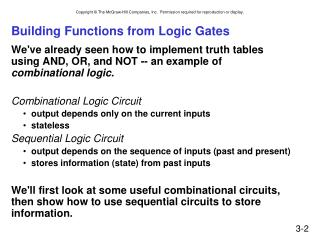Download PresentationBuilding Functions from Logic Gates# Building Functions from Logic Gates - PowerPoint PPT Presentation

Download Presentation##### Building Functions from Logic Gates

Download Policy: Content on the Website is provided to you AS IS for your information and personal use and may not be sold / licensed / shared on other websites without getting consent from its author. While downloading, if for some reason you are not able to download a presentation, the publisher may have deleted the file from their server.

- - - - - - - - - - - - - - - - - - - - - - - - - - - E N D - - - - - - - - - - - - - - - - - - - - - - - - - - -
##### Presentation Transcript

1. Building Functions from Logic Gates • We've already seen how to implement truth tablesusing AND, OR, and NOT -- an example of combinational logic. • Combinational Logic Circuit • output depends only on the current inputs • stateless • Sequential Logic Circuit • output depends on the sequence of inputs (past and present) • stores information (state) from past inputs • We'll first look at some useful combinational circuits,then show how to use sequential circuits to store information.

2. Full Adder Consider computing 7+6=13: A combinational logic design Now, consider one column of this addition:

3. 1-bit Full Adder Truth table for a 1-bit adder: Formulate a circuit for each output

4. The Majority Circuit for CarryOut

5. Odd-Parity Circuit for the Sum

6. Putting It All Together: Full Adder • Add two bits and carry-in, produce one-bit sum and carry-out.

8. Multiplexer (MUX) • A device with multiple inputs and 1 output • Could be used to allocate a resource to one of multiple clients:

9. MUX • A 2n-to-1 multiplexer (MUX) sends one of 2n input lines to a single output line • A MUX has two sets of inputs: • 2n data input lines • n select lines used to pick one of the 2n data inputs • Simplest example is a 2-to-1 MUX

10. 2-to-1 MUX

11. Multiplexer (MUX) • n-bit selector and 2n inputs, one output • output equals one of the inputs, depending on selector 00 01 10 11 4-to-1 MUX

12. Decoder • General example: • Assume that some information is encoded in n bits • For each encoding, we want to activate the (one) correct output line • The general idea: given an n-bit input • Detect which of the 2n combinations is represented • Produce 2n output, only one of which is “1” • A n-to-2n decoder takes an n-bit input and produces 2n outputs. The n inputs represent a binary number that determines which one of the 2n outputs is “true” (i.e., 1).

13. 2-to4 Decoder This circuit decodes a binary input into one of four possible choices, or codes

14. Decoder • n inputs, 2n outputs • exactly one output is 1 for each possible input pattern 2-bit decoder

15. Representing Multi-bit Values • Number bits from right (0) to left (n-1) • just a convention -- could be left to right, but must be consistent • Use brackets to denote range:D[l:r] denotes bit l to bit r, from left to right • May also see A<14:9>, especially in hardware block diagrams. 0 15 A = 0101001101010101 A[2:0] = 101 A[14:9] = 101001

16. Multibit Values in Circuit Diagrams • A 4-to-1 mux, selecting one byte out of a 32-bit value...

17. MUX example -- what does this circuit do?

18. Combinational vs. Sequential • Combinational Circuit • always gives the same output for a given set of inputs • ex: adder always generates sum and carry,regardless of previous inputs • Sequential Circuit • stores information • output depends on stored information (state) plus input • so a given input might produce different outputs,depending on the stored information • example: ticket counter • advances when you push the button • output depends on previous state • useful for building “memory” elements and “state machines”